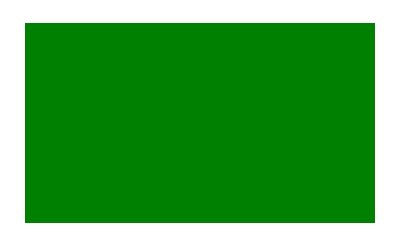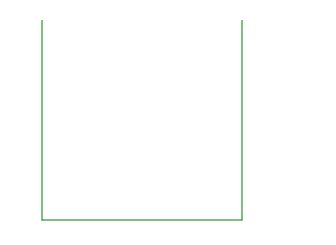Skip to content
Related Articles
HTML | canvas stroke() Method
• Last Updated : 08 Mar, 2021

The canvas stroke() method is used to draw the path you have defined with all those moveTo() and lineTo() methods. The default color of the canvas stroke() method is black.

Syntax:

`context.stroke()`

Example-1:

## html

 ```<``html``>` `<``head``>``    ``<``title``>``        ``HTML canvas stroke() Method``    ````` `<``body``>``    ``<``canvas` `id``=``"GFG"``            ``width``=``"500"``            ``height``=``"300"``>``  ``` `    ``<``script``>``        ``var x =``            ``document.getElementById("GFG");``        ``var contex =``            ``x.getContext("2d");``        ``contex.rect(50, 50, 350, 200);``        ``contex.fillStyle = "green";``        ``contex.fill();``      ` `        ``// Draw the path``        ``contex.stroke();``    ``` `` ``

Output:Example-2:

## html

 ```<``html``>` `<``head``>``    ``<``title``>``        ``HTML canvas stroke() Method``    ````` `<``body``>``    ``<``canvas` `id``=``"GFG"``            ``width``=``"500"``            ``height``=``"300"``>``  ``` `    ``<``script``>``        ``var x =``            ``document.getElementById("GFG");``        ``var contex =``            ``x.getContext("2d");``        ``contex.beginPath();``        ``contex.moveTo(50, 50);``        ``contex.lineTo(50, 250);``        ``contex.lineTo(250, 250);``        ``contex.lineTo(250, 50);``        ``contex.strokeStyle = "green";``        ``contex.stroke();``    ``` `` ``

Output:Supported Browsers:

• Google Chrome
• Internet Explorer 9.0
• Firefox
• Safari
• Opera

My Personal Notes arrow_drop_up